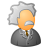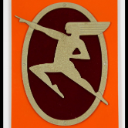# Label appending Expression

739
5
Jump to solution
07-26-2013 07:04 AMNew Contributor
Hey Folks,

I am working on a mapbook and need some assistance with labeling. For parcels in the subdivision I have Rural address shapefile to show the addresses.

In the shapefile I have two main fields; HOUSE ID (String) and ACCESS NUMBER (String). Some of the House ID's are A, B, C while others have numbers, ACCESS field is all numbers. I want to append HOUSE ID and ACCESS NUMBER.

However, here is what I want to do: if the House ID is Alphabetical then it should append after ACCESS NUMBER, if the HOUSE ID is Numeric then it should go before the ACCESS NUMBER.

What would be the function in vbscript or python I would use?

Any help would be greatly appreciated.
Tags (2)
1 Solution

Accepted SolutionsbyMVP Honored Contributor
Ok here is another question.

Lets say some of the HOUSE ID fields are empty, how would you modify the expression to say that if the HOUSE is empty/NULL use ACCESS NUMBER to label?

`Function FindLabel ( [HOUSE], [NUMBER] )   If IsNull( [HOUSE] ) then     Findlabel =  [NUMBER]   Elseif IsNumeric( [HOUSE] ) then     Findlabel =  [HOUSE] & [NUMBER]   Else     FindLabel = [NUMBER] & [HOUSE]   End if End Function`
5 RepliesbyMVP Honored Contributor
You can use this syntax

`Function FindLabel ( [HOUSE], [NUMBER] )  if IsNumeric( [HOUSE] ) then    Findlabel =  [HOUSE] & [NUMBER]  else    FindLabel = [NUMBER] & [HOUSE]  end ifEnd Function`New Contributor
Works great 🙂
Thank you very much!

You can use this syntax

`Function FindLabel ( [HOUSE], [NUMBER] )  if IsNumeric( [HOUSE] ) then    Findlabel =  [HOUSE] & [NUMBER]  else    FindLabel = [NUMBER] & [HOUSE]  end ifEnd Function`New Contributor
Ok here is another question.

Lets say some of the HOUSE ID fields are empty, how would you modify the expression to say that if the HOUSE is empty/NULL use ACCESS NUMBER to label?byMVP Honored Contributor
Ok here is another question.

Lets say some of the HOUSE ID fields are empty, how would you modify the expression to say that if the HOUSE is empty/NULL use ACCESS NUMBER to label?

`Function FindLabel ( [HOUSE], [NUMBER] )   If IsNull( [HOUSE] ) then     Findlabel =  [NUMBER]   Elseif IsNumeric( [HOUSE] ) then     Findlabel =  [HOUSE] & [NUMBER]   Else     FindLabel = [NUMBER] & [HOUSE]   End if End Function`New Contributor
Great.
Thank You! 🙂

`Function FindLabel ( [HOUSE], [NUMBER] )  If IsNull( [HOUSE] ) then    Findlabel =  [NUMBER]  Elseif IsNumeric( [HOUSE] ) then    Findlabel =  [HOUSE] & [NUMBER]  Else    FindLabel = [NUMBER] & [HOUSE]  End ifEnd Function`